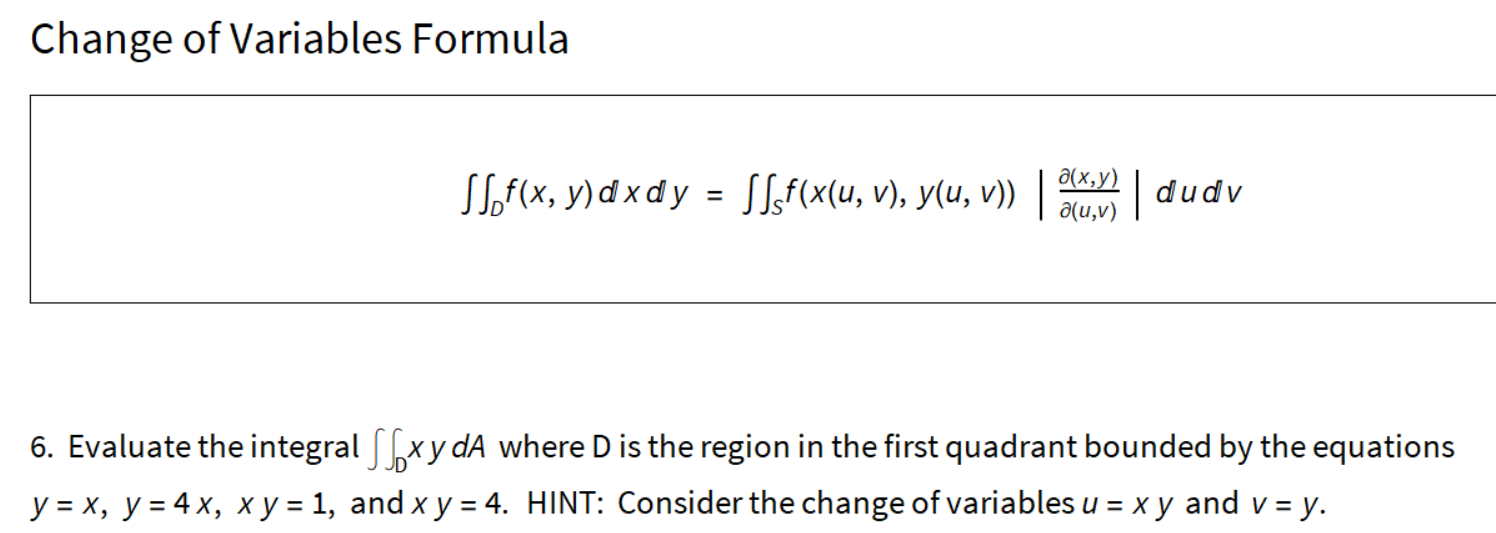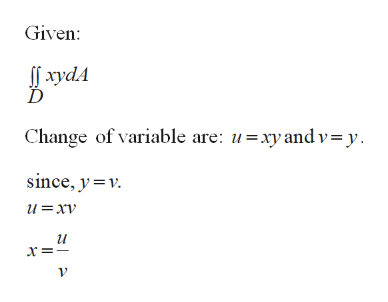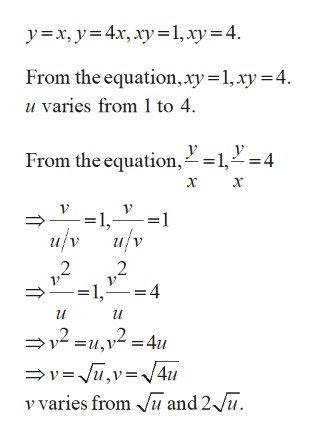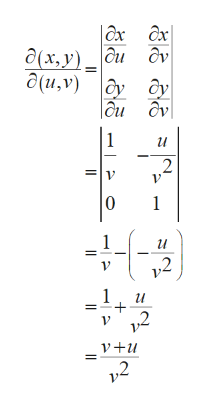# Change of Variables Formulaд(х, у)SSof(x, y) d x d y = SLf(x(u, v), y(u, v))|dudva(u,v)6. Evaluate the integral [xy dA where D is the region in the first quadrant bounded by the equationsy = x, y = 4 x, x y = 1, and x y = 4. HINT: Consider the change of variables u = x y and v = y.

Question
1 views

Evaluate the integral ∫∫ D x y dA where D is the region in the first quadrant bounded by the equations y=x, y=4x, x y=1, and x y=4. HINT: Consider the change of variables u=x y and v=y.help_outlineImage TranscriptioncloseChange of Variables Formula д(х, у) SSof(x, y) d x d y = SLf(x(u, v), y(u, v)) |dudv a(u,v) 6. Evaluate the integral [xy dA where D is the region in the first quadrant bounded by the equations y = x, y = 4 x, x y = 1, and x y = 4. HINT: Consider the change of variables u = x y and v = y. fullscreen
check_circle

star
star
star
star
star
1 Rating
Step 1

Evaluate integral by change of variables.help_outlineImage TranscriptioncloseGiven: If xydA Change of variable are: u=xy and v=y. since, y=v. u=xv и х fullscreen
Step 2

Region bounded by the equation are:help_outlineImage Transcriptionclosey=x, y=4x, xy=1,xy=4. From the equation,xy=1,xy = 4. u varies from 1 to 4. From the equation, 2=1,2=4 х х →=1,- :=1 u/v и/v =1,- и =u,v² =4u =v=Ju,v=J4u v varies from u and 2 Ju. fullscreen
Step 3

Find Jacobian of this ...help_outlineImage Transcriptionclose|x ôx Ô(x,y) _ ĉu ôv бу дy |аи ду д(и, у) и и v2 и v' ,2 v2 fullscreen

### Want to see the full answer?

See Solution

#### Want to see this answer and more?

Solutions are written by subject experts who are available 24/7. Questions are typically answered within 1 hour.*

See Solution
*Response times may vary by subject and question.
Tagged in

### Calculus